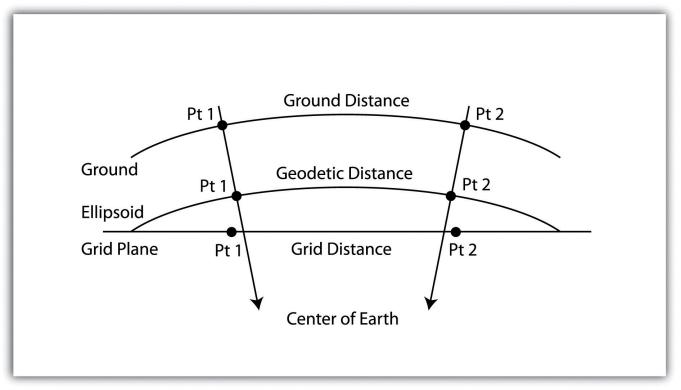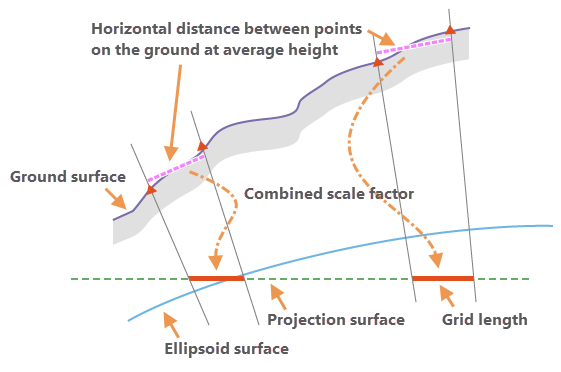What’s the Grid To Ground on Tersus Nuwa

Tersus GNSS has recently developed the latest function, Grid to Ground,coordinate conversion on the Nuwa app. It greatly facilitates the conversion from GNSS survey results to optical survey results and the conversion between grid measurements on map data's projection surface and ground measurement coordinates.

We can see from the picture below that Grid and Ground coordinates are mainly the coordinates located on two different planes. Suppose we have collected some GNSS points using a Tersus receiver, which is grid coordinates. We need to convert all points to ground coordinates. Just find a data point, for example, Pt1, calculate the combined scale factor of its location, apply the parameter to all grid points, and get the ground coordinates.To better understand the potential relationship between the two coordinate systems, we introduce several concepts as follows.

1. Direction offset
Using an optical instrument to measure an unknown point's coordinates with a known point, we only need to obtain two parameters to determine the coordinates. First, the value of the direction of light signal propagation. The second, the distance of light propagation. And in the grid point system and ground point system, the results measured under the two systems are certainly not the same due to the difference in curvature and terrain. The direction offset is the user-defined angle correction, which is applied to the mutual conversion of the direction value under the grid system and the direction angle under the ground coordinate system.

2. Distance factor
Similarly, the direction value has direction offset correction; the propagation distance also has a distance factor that plays a corrective role and is used as a parameter to convert the grid point distance to the ground point distance. And the expression of the distance factor is mainly calculated by the following scale factors.

3. Grid-scale factor
The grid-scale factor is a scale factor that converts between geodetic distance on the ellipsoid surfaceand geodetic distance on the grid plane of projection.It is calculated from the x, y location of the point and the projection surface properties.
Grid distance = Geodetic distance * Grid-scale factor4. Elevation factor
The elevation factor is used to convert the distance between the geodetic distances on the ellipsoid surface and the horizontal ground distances. This factor is calculated from the z elevation of the point.
Geodetic distance = Ground distance * Elevation factor

5. Combined scale factor
The combined scale factor is the final applied value of the distance factor correction and is the main mechanism by which the Tersus Nuwa app implements this function. It can correct for the combined linear distortions due to the curvature of the earth and the ground height of the measured distance above the ellipsoid surface with respect to the projection surface. Combined scale factor = Grid scale factor * Elevation factorThrough the above principle introduction, we can easily understand the operation flow of Nuwa app.

First, we use a GNSS receiver and Nuwa app to complete collecting GNSS point coordinates, which are grid coordinates. Then add a point as the datum point in the Grid to Ground tool of Nuwa app and calculate the combined scale factor of its location, which is also our distance factor, as the conversion factor of Grid and Ground distance.
Grid distance = Ground distance * Combined scale factor

The above equation can be used to calculate the ground distance of each point in the coordinate database with respect to the datum, keeping the original azimuth, and the distance from the datum is the ground distance, so that all the points of the coordinate database can be corrected to get the ground coordinate.

References
1.Ground to grid correction https://pro.arcgis.com/en/pro-app/latest/help/editing/introduction-to-ground-to-grid.htm
2.GNSS & Educational Equations: A Grid-to-Ground Project https://www.xyht.com/surveying/grid-ground-project/
3.Grid vs Ground Distance System https://community.pix4d.com/t/grid-vs-ground-distance-system/3874